#Function Repository Resource:

# GetUnboundSymbols

Get all the non-local symbols that appear in an expression

Contributed by: Richard Hennigan (Wolfram Research)
 ResourceFunction["GetUnboundSymbols"][expr] gets all the non-local symbols that appear in expr. ResourceFunction["GetUnboundSymbols"][expr,wrapper] wraps each symbol in wrapper before returning the result.

## Examples

### Basic Examples

Get the unbound symbols in an expression:

 In:=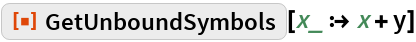Out=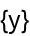Supply a head to be applied to each symbol:

 In:=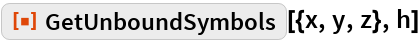Out=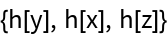Use Unevaluated and a wrapper such as Hold to prevent evaluation:

 In:=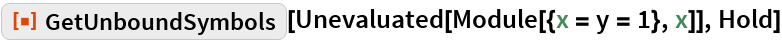Out=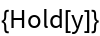In:=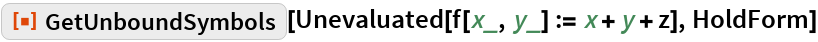Out=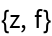### Scope

Ignore symbols that are locally bound by Function:

 In:=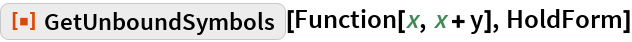Out=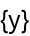GetUnboundSymbols ignores symbols bound by scoping constructs such as Block, With and Module:

 In:=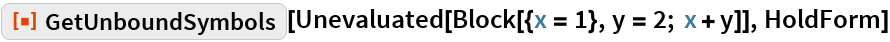Out=In:=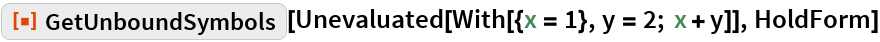Out=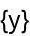In:=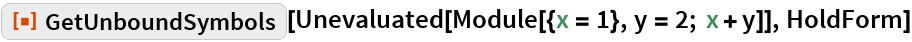Out=RuleDelayed has local bindings:

 In:=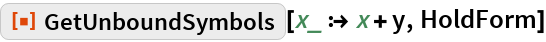Out=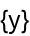SetDelayed also has local bindings:

 In:=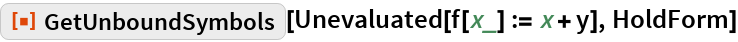Out=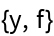The x on the right-hand side of the Set is unbound:

 In:=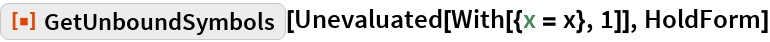Out=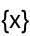### Options

#### ExcludedContexts

Get all unbound symbols regardless of context:

 In:=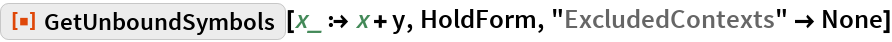Out=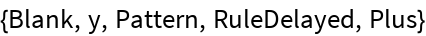Exclude specific contexts:

 In:=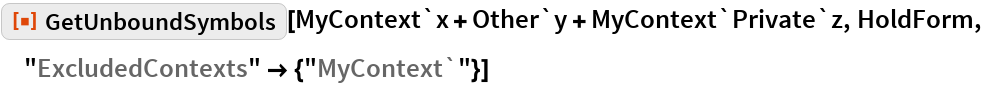Out=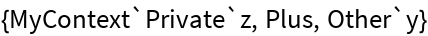Excluded contexts using string patterns:

 In:=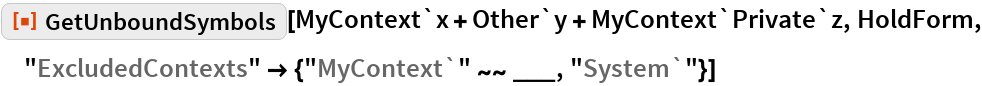Out=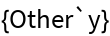Exclude specified contexts in addition to the defaults:

 In:=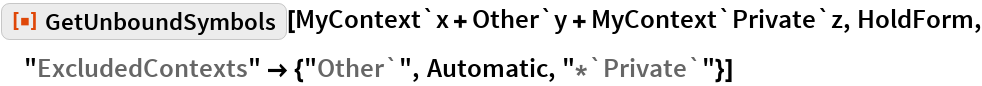Out=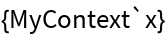### Applications

Get dependent symbols in a definition while excluding local symbols:

 In:=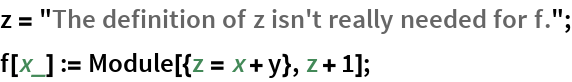In:=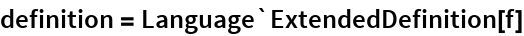Out=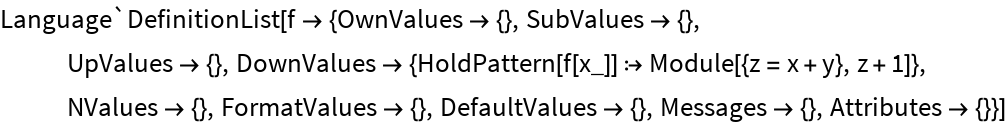In:=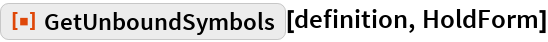Out=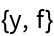### Possible Issues

GetUnboundSymbols does not predict future scoping:

 In:=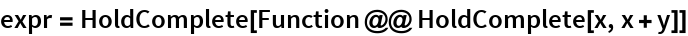Out=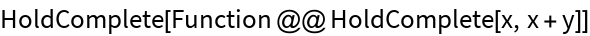In:=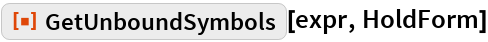Out=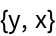When evaluated, x becomes a local symbol:

 In:=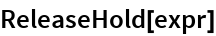Out=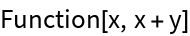In:=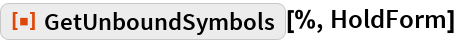Out=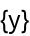## Requirements

Wolfram Language 11.3 (March 2018) or above